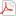Reference : Integer Programs with Prescribed Number of Solutions and a Weighted Version of Doigno...
 Document type : Scientific journals : Article Discipline(s) : Physical, chemical, mathematical & earth Sciences : Mathematics To cite this reference: http://hdl.handle.net/2268/166141
 Title : Integer Programs with Prescribed Number of Solutions and a Weighted Version of Doignon-Bell-Scarf’s Theorem Language : English Author, co-author : Aliev, Iskander [Cardiff University > Department of Mathematics > > >] De Loera, Jesus [University of California, Davis - UC Davis > Department of Mathematics > > >] Louveaux, Quentin[Université de Liège - ULiège > Dép. d'électric., électron. et informat. (Inst.Montefiore) > Système et modélisation : Optimisation discrète >] Publication date : Jun-2014 Journal title : Lecture Notes in Computer Science Publisher : Springer Special issue title : Integer Programming and Combinatorial Optimization Peer reviewed : Yes Audience : International ISSN : 0302-9743 e-ISSN : 1611-3349 City : Berlin Country : Germany Keywords : [en] Integer Programming Abstract : [en] In this paper we study a generalization of the classical fesibility problem in integer linear programming, where an ILP needs to have a prescribed number of solutions to be considered solved. We first provide a generalization of the famous Doignon-Bell-Scarf theorem: Given an integer k, we prove that there exists a constant c(k, n), depending only on the dimension n and k, such that if a polyhedron {x : Ax ≤ b} contains exactly k integer solutions, then there exists a subset of the rows of cardinality no more than c(k,n), defining a polyhedron that contains exactly the same k integer solutions. The second contribution of the article presents a structure theory that characterizes precisely the set Sg≥k (A) of all vectors b such that the problem Ax = b, x ≥ 0, x ∈ Zn , has at least k-solutions. We demonstrate that this set is finitely generated, a union of translated copies of a semigroup which can be computed explicitly via Hilbert bases computation. Similar results can be derived for those right-hand-side vectors that have exactly k solutions or fewer than k solutions. Finally we show that, when n, k are fixed natural numbers, one can compute in polynomial time an encoding of Sg≥k(A) as a generating function, using a short sum of rational functions. As a consequence, one can identify all right-hand-side vectors that have exactly k solutions (similarly for at least k or less than k solutions). Under the same assumptions we prove that the k-Frobenius number can be computed in polynomial time. Permalink : http://hdl.handle.net/2268/166141

File(s) associated to this reference

Fulltext file(s):

FileCommentaryVersionSizeAccessOpen access
reallyFinalIPCO-ilps-with-k-solutions.pdfPublisher postprint371.98 kBView/OpenAll documents in ORBi are protected by a user license.